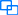线性表面试题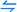排序面试题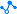二叉树面试题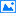图算法面试题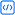高频算法面试题

# 线性表面试题

1)单向链表，双向链表

2)带头链表，不带头链表
3)循环的，非循环的

## 003枚举法创建链表？

``````class ListNode {
int val;
ListNode next;
public ListNode(int val) {
this.val = val;
}
}``````

``````public ListNode head;//链表的头
public void creatList(){
ListNode listNode1 = new ListNode(11);
ListNode listNode2 = new ListNode(22);
ListNode listNode3 = new ListNode(33);
ListNode listNode4 = new ListNode(44);

listNode1.next = listNode2;
listNode2.next = listNode3;
listNode3.next = listNode4;
}
``````

## 004尾插法创建链表

``````class ListNode {
int val;
ListNode next;
public ListNode(int val) {
this.val = val;
}
}``````

``````public ListNode head;//链表的头
ListNode node = new ListNode(data);
}else {
while(cur.next != null){
cur = cur.next;
}
cur.next = node;
}
}``````

## 005如何打印链表？

``````public void display(){
while(cur != null){
System.out.print(cur.val+" ");
cur = cur.next;
}
System.out.println();
}``````

## 006通过栈将链表逆序输出，如链表为1->2->3，打印出3 2 1

``````/**
* 栈
*/
public List<Integer> printListFromQueue(ListNode listNode) {
Stack<Integer> stack = new Stack<>();
while (listNode != null) {
listNode = listNode.next;
}
List<Integer> list = new ArrayList<>();
while (!stack.isEmpty()) {
}
return list;
}``````

## 007通过递归将链表逆序输出，如链表为1->2->3，打印出3 2 1。

``````/**
* 递归
*/
public List<Integer> printListFromDG(ListNode listNode) {
List<Integer> list = new ArrayList<>();
if (listNode != null) {
}
return list;
}``````

## 009用全部压栈法判断链表是否为回文结构。

``````public boolean isPalindrome(ListNode head) {
Stack<Integer> stack = new Stack();
//把链表节点的值存放到栈中
while (temp != null) {
stack.push(temp.val);
temp = temp.next;
}
//然后再出栈
return false;
}
}
return true;
}``````

## 010用部分压栈法判断链表是否为回文结构。

``````public boolean isPalindrome(ListNode head) {
return true;
Stack<Integer> stack = new Stack();
//链表的长度
int len = 0;
//把链表节点的值存放到栈中
while (temp != null) {
stack.push(temp.val);
temp = temp.next;
len++;
}
//len长度除以2
len >>= 1;
//然后再出栈
while (len-- >= 0) {
return false;
}
return true;
}``````

## 011用快慢指针法法判断链表是否为回文结构。

``````public boolean isPalindrome(ListNode head) {
return true;
}
ListNode pre = head, prepre = null;
while(fast != null && fast.next != null) {
pre = slow;
slow = slow.next;
fast = fast.next.next;
//将前半部分链表反转
pre.next = prepre;
prepre = pre;
}
if(fast != null) {
slow = slow.next;
}
while(pre != null && slow != null) {
if(pre.val != slow.val) {
return false;
}
pre = pre.next;
slow = slow.next;
}
return true;
}``````

## 012利用HashSet判断链表是否有环？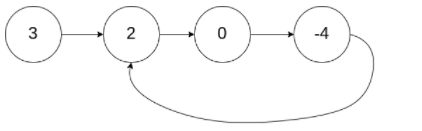``````public static boolean hasCycle1(ListNode head){
//存入的是ListNode类型而不是值
HashSet<ListNode> hashSet = new HashSet<>();
return true;
}
}
return false;
}``````

## 013利用快慢指针判断链表是否有环？

``````public static boolean hasCycle2(ListNode head){
return false;
}
//定义两个指针,快指针比慢指针多一步

while (slow!=fast){
if (fast==null||fast.next==null){
return false;
}
//快指针比慢指针多一步
slow=slow.next;
fast=fast.next.next;
}
return true;
}``````

## 014合并两个有序链表

``````public ListNode mergeTwoLists(ListNode l1, ListNode l2) {
if(l1 == null) return l2;
if(l2 == null) return l1;
if(l1.val < l2.val){
l1.next = mergeTwoLists(l1.next,l2);
return l1;
}else{
l2.next = mergeTwoLists(l1,l2.next);
return l2;
}
} ``````

## 015删除排序链表中的重复元素。

``````//一次遍历，注意边界条件。
while(cur != null && cur.next != null){
if(cur.val == cur.next.val)
cur.next = cur.next.next;
else
cur = cur.next;
}
} ``````

## 016删除链表的倒数第N个节点。

``````//设置哑节点1，让它走 n+1 步，再设置哑节点2，然后哑节点1和哑节点2一起移动，
//直到哑节点1走完链表，此时哑节点1和哑节点2之间正好隔着 n 个节点，再通过哑节点2删除倒数第 n 个节点。
public ListNode removeNthFromEnd(ListNode head, int n) {
ListNode dummy = new ListNode(0);
ListNode first = dummy;
for (int i = 1; i <= n + 1;i++){
first = first.next;
}
ListNode second = dummy;
while(first != null){
first = first.next;
second = second.next;
}
second.next = second.next.next;
return dummy.next;
} ``````

## 017两两交换链表中的节点.

``````//设置哑节点，注意循环条件，指针移动的速度是2（因为需要两两交换节点）。
public ListNode swapPairs (ListNode head) {
ListNode dummy = new ListNode(-1);
ListNode pre = dummy;
while (pre.next != null && pre.next.next != null) {
ListNode l1 = pre.next;
ListNode l2 = pre.next.next;
l1.next = l2.next;
l2.next = l1;
pre.next = l2;
pre = l1;
}
return dummy.next;
} ``````

## 018两数相加

``````//既然不能改变链表的结构（翻转链表），那就用一个栈来保存链表中的值，可以做到逆向输出。
//相加部分的代码逻辑就按常规思路写。
public ListNode addTwoNumbers (ListNode l1, ListNode l2) {
Stack<Integer> l1Stack = listNodetoStack(l1);
Stack<Integer> l2Stack = listNodetoStack(l2);
int carry = 0;
while (!l1Stack.isEmpty() || !l2Stack.isEmpty() || carry != 0) {
int x = l1Stack.isEmpty() ? 0 : l1Stack.pop();
int y = l2Stack.isEmpty() ? 0 : l2Stack.pop();
int sum = x + y + carry;
ListNode node = new ListNode(sum % 10);
carry = sum / 10;
}
}
private Stack<Integer> listNodetoStack (ListNode head) {
Stack<Integer> stack = new Stack<>();
}
return stack;
}
``````

## 019分隔链表

● root 的长度范围： [0, 1000].
● 输入的每个节点的大小范围：[0, 999].
● k 的取值范围： [1, 50].

``````/*思路：先统计出链表长度，除以 k, 求商和余数，其中：
● 余数代表最后结果中有多少个长链表
● 商代表每个短链表的长度（结果集中后部的链表）
● 长链表比短链表多一个节点*/
public ListNode[] splitListToParts (ListNode root, int k) {
ListNode cur = root;
int len = 0;
while (cur != null) {
cur = cur.next;
len++;
}
int mod = len % k;
int size = len / k;
cur = root;
ListNode[] ans = new ListNode[k];
for (int i = 0; cur != null && i < k; i++) {
ans[i] = cur;
int curSize = size + (mod-- > 0 ? 1 : 0);
for (int j = 0; j < curSize - 1; j++) {
cur = cur.next;
}
ListNode next = cur.next;
cur.next = null;
cur = next;
}
return ans;
} ``````

## 020奇偶链表

● 应当保持奇数节点和偶数节点的相对顺序。
● 链表的第一个节点视为奇数节点，第二个节点视为偶数节点，以此类推。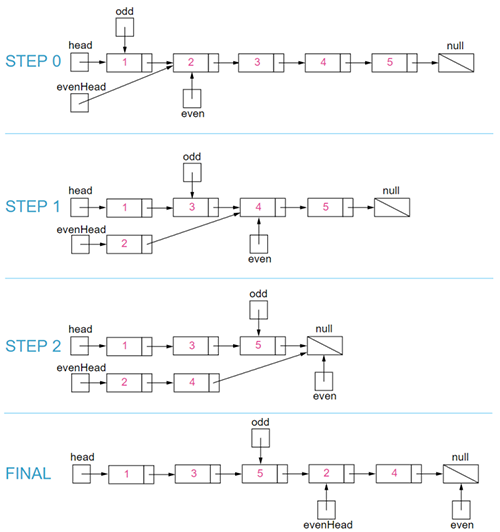``````public ListNode oddEvenList (ListNode head) {
while (even != null && even.next != null) {
odd.next = even.next;
odd = odd.next;
even.next = odd.next;
even = even.next;
}
}``````

## 021什么是栈？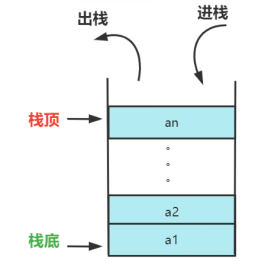## 022栈的基本使用

``````public static void main(String[] args) {
Stack < Integer > stack = new Stack < Integer > ();
//入栈
stack.push(3); //栈底
stack.push(4);
stack.push(5);
stack.push(7); //栈顶
//出栈：弹出栈顶元素
System.out.println(stack.pop()); //7
//再弹一次，此时栈顶元素为5了，如下。
System.out.println(stack.pop());
//获取栈顶元素但不删除,这时的栈顶元素以及是4了
System.out.println(stack.peek());
//判断栈顶元素是否为空
System.out.println(stack.empty());
Stack < Integer > stack1 = new Stack < > ();
System.out.println(stack1.empty());
//获取栈中的元素的位置，栈顶为1号，此时stack中有3,4两个元素，所以4元素的位置为1号
System.out.println(stack.search(4));
//使用父类的方法，stack继承自Vector
System.out.println(stack.isEmpty());
}``````

A. 栈顶元素最先能被删除
B. 栈顶元素最后才能被删除
C. 栈底元素永远不能被删除
D. 栈底元素最先被删除

A. 2 3 4 1 5
B. 5 4 1 3 2
C. 2 3 1 4 5
D. 1 5 4 3 2

A. 栈的顶
B. 栈的底
C. 栈指针
D. 栈中的数据

A. 堆区，栈区
B. 常量区，堆区
C. 全局区，栈区
D. 栈区，堆区

A. SSSSXXXX
B. SSSXXSXX
C. SXSSXXSX
D. SXSXSXSX

A. XYZ
B. YZX
C. ZXY
D. ZYX

## 029设计一个获取栈中最小元素的栈

``````class MinStack {
private Stack < Integer > stack;
private Stack < Integer > minStack;

public MinStack() {
stack = new Stack < > ();
minStack = new Stack < > ();
}

public void push(int val) {
stack.push(val);
if (!minStack.empty()) {
int top = minStack.peek();
if (val <= top) {
minStack.push(val);
}
} else {
minStack.push(val);
}
}

public void pop() {
int popVal = stack.pop();
if (!minStack.empty()) {
int top = minStack.peek();
if (top == popVal) {
minStack.pop();
}
}
}

public int top() {
return stack.peek();
}

public int getMin() {
return minStack.peek();
}
}``````

## 030判断某个数组是否是正确的出栈顺序。

``````public class Demo5 {
public static void main(String[] args) {
int[] A = {
1, 2, 3, 4, 5
};
int[] B = {
4, 5, 3, 2, 1
};
System.out.println(IsPopOrder(A, B));
}
public static boolean IsPopOrder(int[] pushA, int[] popA) {
Stack < Integer > stack = new Stack < > ();
int j = 0;
for (int i = 0; i < pushA.length; i++) {
stack.push(pushA[i]);
while (j < popA.length && !stack.empty() && stack.peek() == popA[j]) {
stack.pop();
j++;
}
}
return stack.empty();
}
}``````

## 031什么是队列？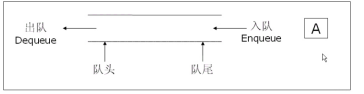## 032一个队列的进队顺序是1,2，....n，若进队和出队可以交替进行，则出队顺序可能是( )。

A. 1,2,........,n
B. 1,2,4,3,5,6,.....,n
C. n,n-1,....,1
D. 以上均有可能

## 033队列的“先进先出”特性是指( )。

A. 最早插入队列中的元素总是最后被删除
B. 当同时进行插入、删除操作时，总是插入操作优先
C. 每当有删除操作时，总是要先做一次插入操作
D. 每次从队列中删除的总是最早插入的元素

B选项中，插入和删除的操作看谁先执行就就优先操作；
C选项中，删除操作时和插入没有关系；

## 034循环队列的出队操作为（）。

A.sq.front=(sq.front+1)% maxsize；
B.sq.front=sq.front+1；
C.sq.rear=(sq.rear+1)% maxsize；
D.sq.rear=sq.rear+1；

## 035队列是一种运算受限的线性表，以下说法准确的是？

A.单向队列在允许删除的一端叫队头，在允许插入的一端叫队尾。
B.单向队列在允许删除的一端叫队尾，在允许插入的一端叫队头。
C.队列可以用数组实现，也可以用链表实现
D.队列是先进先出的，栈是后进先出的

## 036下述有关栈和队列的区别，说法错误的是？

A.栈是限定只能在表的一端进行插入和删除操作。
B.队列是限定只能在表的一端进行插入和在另一端进行删除操作。
C.栈和队列都属于线性表
D.栈的插入操作时间复杂度都是o(1)，队列的插入操作时间复杂度是o(n)

## 037数组实现队列？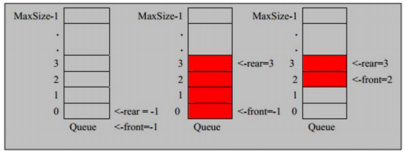1)将尾指针往后移： rear+1 , 当 front == rear 【空】
2)若尾指针 rear 小于队列的最大下标 maxSize-1 ，则将数据存入 rear 所指的数组元素中，否则无法存入数据。rear == maxSize - 1[ 队列满 ]

## 038数组实现队列的代码实现。

``````import java.util.Scanner;
public class ArrayQueueDemo {
public static void main(String[] args) {
//测试一把
// 创建一个队列
ArrayQueue queue = new ArrayQueue(3);
char key = ' '; //接收用户输入
Scanner scanner = new Scanner(System.in); //
boolean loop = true;
//输出一个菜单
while (loop) {
System.out.println("s(show): 显示队列");
System.out.println("e(exit): 退出程序");
System.out.println("g(get): 从队列取出数据");
key = scanner.next().charAt(0); //接收一个字符
switch (key) {
case 's':
queue.showQueue();
break;
case 'a':
System.out.println("输出一个数");
int value = scanner.nextInt();
break;
case 'g': //取出数据
try {
int res = queue.getQueue();
System.out.printf("取出的数据是%d\n", res);
} catch (Exception e) {
// TODO: handle exception
System.out.println(e.getMessage());
}
break;
case 'h': //查看队列头的数据
try {
System.out.printf("队列头的数据是%d\n", res);
} catch (Exception e) {
// TODO: handle exception
System.out.println(e.getMessage());
}
break;
case 'e': //退出
scanner.close();
loop = false;
break;
default:
break;
}
}
System.out.println("程序退出~~");
}
}
class ArrayQueue {
private int maxSize; //表示数组的最大容量
private int front; //表示队列头
private int rear; //表示队列尾
private int[] arr; //该数组用于存放数据，模拟队列

//创建队列的构造器
public ArrayQueue(int arrMaxSize) {
maxSize = arrMaxSize;
arr = new int[maxSize];
front = -1; // 指向队列头部前一个位置
rear = -1; // 指向队列尾，指向队列尾的数据(即就是队列最后一个数据)
}

//判断队列是否满
public boolean isFull() {
return rear == maxSize - 1;
}

// 判断队列是否为空
public boolean isEmpty() {
return rear == front;
}

// 添加数据到队列
// 判断队列是否满
if (isFull()) {
System.out.println("队列满，不能加入数据~");
return;
}

rear++; // 让 rear 后移
arr[rear] = n;
}

// 获取队列的数据, 出队列
public int getQueue() {
// 判断队列是否空
if (isEmpty()) {
// 通过抛出异常
throw new RuntimeException("队列空，不能取数据");
}
front++; // front 后移
return arr[front];
}

// 显示队列的所有数据
public void showQueue() {
// 遍历
if (isEmpty()) {
System.out.println("队列空的，没有数据~~");
return;
}

for (int i = 0; i < arr.length; i++) {
System.out.printf("arr[%d]=%d\n", i, arr[i]);
}
}

// 显示队列的头数据， 注意不是取出数据
// 判断
if (isEmpty()) {
throw new RuntimeException("队列空的，没有数据~~");
}
return arr[front + 1];
}
}``````

## 039打印数组

``````数组为：{1,2,3,4,5}

``````package com.bjpowernode.array;
/*创建一个长度为6的整数数组，数组中有六个整数(直接赋值即可)。遍历数组中的每个元素，元素之间用空格隔开。
比如数组为：{1,2,3,4,5}
打印结果：1 2 3 4 5*/
public class Test1 {
public static void main(String[] args) {
int [] arr={1,2,3,4,5};//定义整数类型数组
for(int i=0;i<arr.length;i++){
System.out.print(arr[i]+"\t");
}
}
}
``````

## 040打印数组最小值

``````package com.bjpowernode.array;
/*

### 训练提示
1. 数组的元素是小数，需要定义小数类型数组。
2. 找最小值和找最大值的思路是一样的。*/
public class Test2 {
public static void main(String[] args) {
double[] arr={12.9,53.54,75.0,99.1,3.14};//定义一个double数组
double min=arr;
for(int i=1;i< arr.length;i++){
if(min>arr[i]){
min=arr[i];
}
}
System.out.println("最小值是"+min);
}
}``````

A. 520
B. 522
C. 524
D. 518

A. 12,18,14,16
B. 12,14, 18,16
C. 6,9,7,8
D. 6,7,9,8

A. a[2*1]
B. a
C. a[4-2]
D. a[2+2]